Example Questions

Example Question #4 : Acute / Obtuse Isosceles Triangles

Points A and B lie on a circle centered at Z, where central angle <AZB measures 140°. What is the measure of angle <ZAB?

20°

30°

Cannot be determined from the given information

15°

25°

20°

Explanation:

Because line segments ZA and ZB are radii of the circle, they must have the same length. That makes triangle ABZ an isosceles triangle, with <ZAB and <ZBA having the same measure. Because the three angles of a triangle must sum to 180°, you can express this in the equation:

140 + 2x = 180 --> 2x = 40 --> x = 20

Example Question #5 : Acute / Obtuse Isosceles Triangles

Triangle FGH has equal lengths for FG and GH; what is the measure of F, if G measures 40 degrees?

40 degrees

100 degrees

140 degrees

70 degrees

70 degrees

Explanation:

It's good to draw a diagram for this; we know that it's an isosceles triangle; remember that the angles of a triangle total 180 degrees.

Angle G for this triangle is the one angle that doesn't correspond to an equal side of the isosceles triangle (opposite side to the angle), so that means F = H, and that F + H + 40 = 180,

By substitution we find that F * 2 = 140 and angle F = 70 degrees.

Example Question #6 : Acute / Obtuse Isosceles Triangles

The vertex angle of an isosceles triangle is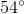.  What is the base angle?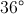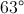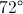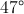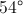Explanation:

An isosceles triangle has two congruent base angles and one vertex angle.  Each triangle contains.  Let= base angle, so the equation becomes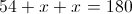.  Solving forgives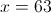Example Question #7 : Acute / Obtuse Isosceles Triangles

In an isosceles triangle the base angle is five less than twice the vertex angle.  What is the sum of the vertex angle and the base angle?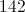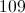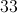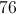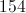Explanation:

Every triangle has 180 degrees.  An isosceles triangle has one vertex angle and two congruent base angles.

Let= the vertex angle

and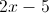= base angle

So the equation to solve becomes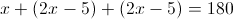or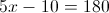Thus the vertex angle is 38 and the base angle is 71 and their sum is 109.

Example Question #8 : Acute / Obtuse Isosceles Triangles

Sides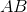and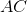in this triangle are equal. What is the measure of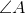?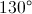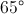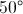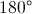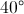Explanation:

This triangle has an angle of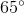. We also know it has another angle ofat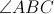because the two sides are equal. Adding those two angles together gives us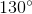total. Since a triangle hastotal, we subtract 130 from 180 and get 50.

Example Question #1 : How To Find An Angle In An Acute / Obtuse Isosceles Triangle

An isosceles triangle has a base angle that is six more than three times the vertex angle.  What is the base angle?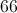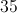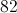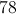Explanation:

Every triangle has 180 degrees.  An isosceles triangle has one vertex angle and two congruent base angles.

Let= vertex angle and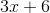= base angle.

Then the equation to solve becomes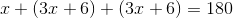or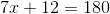.

Solving forgives a vertex angle of 24 degrees and a base angle of 78 degrees.

Example Question #2 : How To Find An Angle In An Acute / Obtuse Isosceles Triangle

The base angle of an isosceles triangle is thirteen more than three times the vertex angle. What is the difference between the vertex angle and the base angle?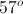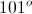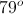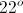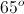Explanation:

Every triangle has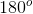. An isosceles triangle has one vertex ange, and two congruent base angles.

Letbe the vertex angle and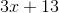be the base angle.

The equation to solve becomes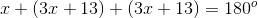, since the base angle occurs twice.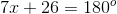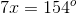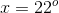Now we can solve for the vertex angle.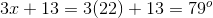The difference between the vertex angle and the base angle is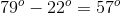.

Example Question #3 : How To Find An Angle In An Acute / Obtuse Isosceles Triangle

A particular acute isosceles triangle has an internal angle measuring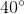. Which of the following must be the other two angles?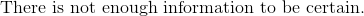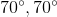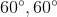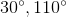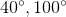Explanation:

By definition, an acute isosceles triangle will have at least two sides (and at least two corresponding angles) that are congruent, and no angle will be greater than. Addtionally, like all triangles, the three angles will sum to. Thus, of our two answers which sum to, onlyis valid, aswould violate the "acute" part of the definition.

Example Question #4 : How To Find An Angle In An Acute / Obtuse Isosceles Triangle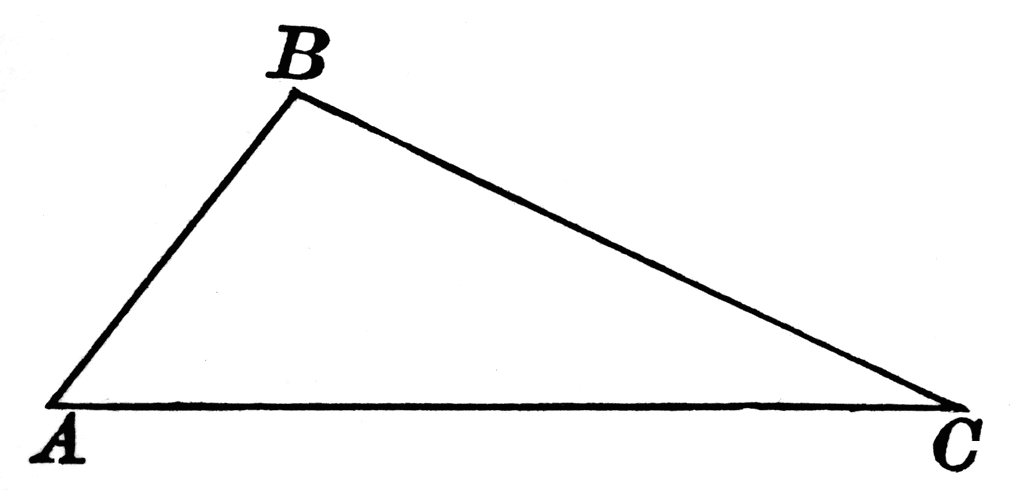In triangle ABC, Angle A = x degrees, Angle B = 2x degrees, and Angle C = 3x+30 degrees. How many degrees is Angle B?

50°

105°

45°

25°

30°

50°

Explanation:

Because the interior angles of a triangle add up to 180°, we can create an equation using the variables given in the problem: x+2x+(3x+30)=180. This simplifies to 6X+30=180. When we subtract 30 from both sides, we get 6x=150. Then, when we divide both sides by 6, we get x=25. Because Angle B=2x degrees, we multiply 25 times 2. Thus, Angle B is equal to 50°. If you got an answer of 25, you may have forgotten to multiply by 2. If you got 105, you may have found Angle C instead of Angle B.

Example Question #11 : Isosceles Triangles

A triangle has a perimeter of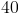inches with one side of lengthinches. If the remaining two sides have lengths in a ratio of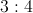, what is length of the shortest side of the triangle?The answer is.
Since we know that the permieter isinches and one side isinches, it can be determined that the remaining two sides must combine to be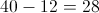inches. The ratio of the remaining two sides iswhich means 3 parts : 4 parts or 7 parts combined. We can then set up the equation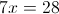, and divide both sides bywhich means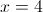. The ratio of the remaining side lengths then becomes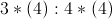or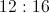. We now know the 3 side lengths are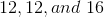.is the shortest side and thus the answer.